### Introduction

In this exercise we will be going through some very introductory steps for using R effectively. We will read in, manipulate, analyze and export data. We will be using RStudio which is a user friendly graphical interface to R. Please be aware that R has an extremely diverse developer ecosystem and is a very function rich tool. The steps used to complete each step of this exercise can be completed in a variety of ways. The steps shown here just demonstrate one possible solution.

Important to remember! You can get help with any R function while in R! This can be done by typing a ? ahead of the command:

`?read.table`

Additionally, the internet has a large number of useful resources:

In this exercise we will be looking at and analyzing data in a “data frame”. A data frame is basically R’s table format. The data frame we will be using is a mock metagenome quantifying the abundance of 30 classes of bacteria in healthy or sick individuals. The context of the data is not important. The goal of this exercise is to familiarize you with working with data in R, and tabular data is something that R excels at manipulating and analyzing, so the lessons learned working with this data set should be extendable to a variety of uses.

### Step 1: Getting data into R

You should first set your working directory (setwd) to the location of the example file called “metagenome_R_example.txt“:

For example:

`setwd("~/Desktop/Data/R/")`

Then you should use the read.table function to read this file into RStudio.

We will be calling this data table “bac” which is short for bacteria. You can name files whatever you want, but simplicity is encouraged.

`bac <- read.table("metagenome_R_example.txt")`

Note that when a file outside of R is referenced it must appear in quotes. Go ahead and take a look at the data table by simply typing bac.

You should see the full data table spill out on the screen. Since this data table is large it will be difficult to look at in its entirety, fortunately we can use some basic commands to view small slices of the full data table. You can slice data using the following convention:

data_file[rows,columns]

The rows and columns can be separated by a : to describe a range. For example, if we just wanted to look at the first 3 rows of a our data file we would type:

>bac[1:3,]

To look at the first three columns we would type:

>bac[,1:3]

Note the importance of the placement of the comma.

We can now use the head command (type ?head to get an idea of what it does) to access a selected portion of our data table.

### Activity

Exercise 1: Look at the first few rows of the bac data table using the head function:

You should spend some time slicing the data table up in various ways.

You can specify a column of data using the \$ before the column name. Try defining the Clostridia column using \$Clostridia. For simplicity, specify the first 10 rows.

[toggle hide=”yes” border=”yes” style=”white”]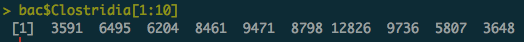[/toggle]

You should become comfortable with defining subsets of the data table  before moving forward. Please spend some time defining various subsets of the data table and observing the output.

Exercise 2: Creating new data tables from pre-existing data tables.

You can create new data tables with subsets of the original data table. You do this by assigning a subset of data using <-. The basic convention for creating a new data table (or any other data structure) is:

new_file <- old_file(functions)

For example, if we wanted to create a new data table with just Clostridia in it we would do the following:

clostridia_only <- bac\$Clostridia

Create a new data table with just the descriptive data of “sex” and “sickness”. Open the data file by typing it’s name at the command prompt to confirm it is what you expected it to be.

Exercise 3: Use the summary function on the descriptive data to quickly quantify each type of sample in the data table.

The summary function is quite useful and a great tool that does precisely what it sounds like. It summarizes the given data and provides basic metrics and statistics. Produce a summary of the bac data table. Be aware that it is going to summarize every column in the data table and produce a relatively long output. You will need to scroll up to get information about the first columns in the data table.

You can now see that we have equal numbers of “Sick” and “Healthy” samples, but a disproportionate partition amongst the sexes. You can also quickly view the basic structure, including min/max, mean and quartiles of the data in each column of the data table.

Exercise 3: Basic plotting.

Boxplots in R use the conventions detailed in the figure below and are useful for describing numerical data in a column.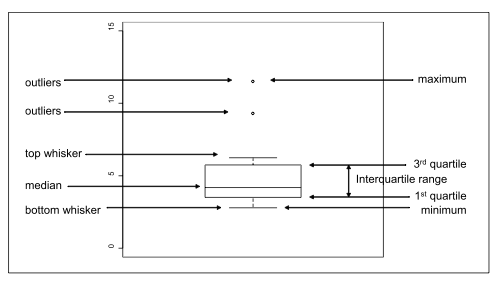Create a boxplot comparing the levels of Clostridia between healthy and sick individuals. Note, this is one of those occasions were there are a variety of ways to accomplish the task. One simple way to discern between healthy and sick is by defining them by rows. In our case, all of the sick samples are in [1:20] and healthy in [21:40]. Try to create the plot below using the boxplot function.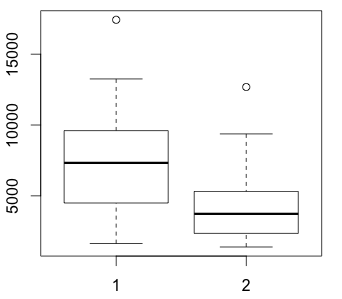[toggle hide=”yes” border=”yes” style=”white”]>boxplot(bac\$Clostridia[1:20], bac\$Clostridia[21:40])[/toggle]

Notice how this boxplot doesn’t have a lot of titles or other information on it. Let’s do some manipulations to this graph to try and make it a little easier to read.

First, plot the boxplots on a log scale using the log function to transform the data.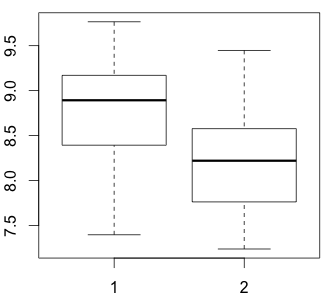[toggle hide=”yes” border=”yes” style=”white”] >boxplot(log(bac\$Clostridia[1:20]), log(bac\$Clostridia[21:40]))[/toggle]

This helps normalize the plots, but we are still lacking a lot of basic descritpive information. To fix this, let’s add some titles, better axis descriptions and some visual enhancements. Do the following to the graph:

– Add a main title called “Comparison of Clostridia between Healthy and Sick Individuals”

– Add a y-axis using ylab called “Log10 Clostridia”

– Add “Healthy” and “Sick” labels to the x-axis. This one is a bit tricky and you have to use the names function in box plots. Use the ?boxplot help page for assistance and remember that text strings should be enclosed in quotes.

– Color the box plots light grey

– Increase the line width (lwd) of the box plots to 2

The final graph should look something like this:Most of the functions to modify are easy to understand. For example, you use ylab to label the y-axis. However, labeling the x-axis is done a bit differently. You have to define each bar plot which requires the names attribute. If you are having problems with this you can toggle the answer below.

[toggle hide=”yes” border=”yes” style=”white”]>boxplot(log(bac\$Clostridia[1:20]), log(bac\$Clostridia[21:40]), main=”Comparison of Clostridia between Healthy and Sick Individuals”, ylab=”log10 Clostridia”, names=c(“Healthy”, “Sick”), lwd=2, col=”light grey”)[/toggle]

Another frequently used plot in R is plotting one set of data against another. This can be performed simply using the plot function. Try this out by plotting the levels of BacilliClostridia and Bacteroida against one another using the following command:

>plot(bac[,30:32])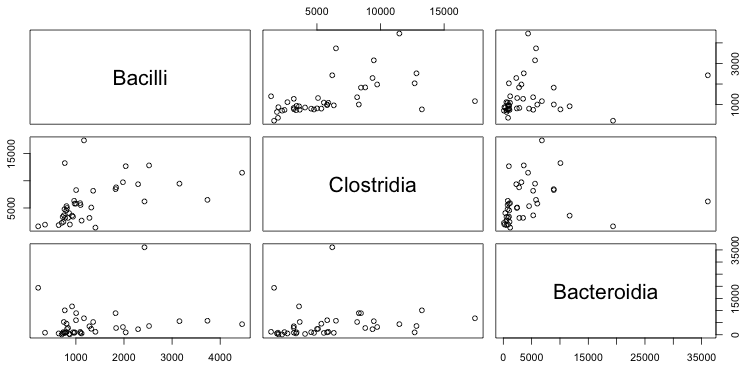These plots are easy to generate and are useful for finding associations between distinct sets of data.

Exercise 4: Defining layouts

R has powerful graphical layout tools. These layout options allow you to plot several graphs next to one another in a very controlled manner. There are a variety of ways to define these layouts, but the simplest and most frequently used way is to define the layout paramaters using the par function.

For example, the following command will define a 2×2 layout for graphing:

>par(mfrow=c(2,2))

While this would define a single row with three columns (1×3)

>par(mfrow=c(1,3))

These settings are maintained by R until you change them. To get back to the default layout you can simply enter:

>par(mfrow=c(1,1))

Define a 1×3 layout and plot three different box plots using the Clostridia data we have already been working with. For something very simple, lets see how taking the log and sqrt of the data alter the structure of the data. You should be able to generate these three plots to obtain the following: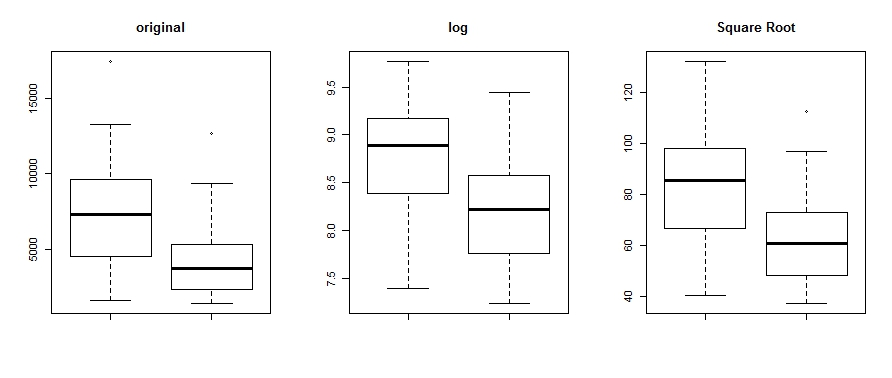[toggle hide=”yes” border=”yes” style=”white”]>par(mfrow=c(1,3))
boxplot(bac\$Clostridia[1:20], bac\$Clostridia[21:40], main=”original”)
boxplot(log(bac\$Clostridia[1:20]), log(bac\$Clostridia[21:40]), main=”log”)
boxplot(sqrt(bac\$Clostridia[1:20]), sqrt(bac\$Clostridia[21:40]), main=”Square Root”)[/toggle]

Exercise 5: Basic Statistics

R has an extensive built-in library of statistical tests that can be performed on data. In addition, their are a large number of libraries specific for various statistical analysis. In this exercise, we will be performing basic T-tests just to get you familiar with the syntax required of statistical analysis.

The function t.test performs either a one or two sample Student’s test. Using this command you should be able to determine if their is a statistically significant difference in the amounts of various bacteria in healthy and sick individuals.

In previous exercises we defined groups of sick/healthy by their rows (ie. healthy was bac[21:40,]. However, there is a simpler way to do this which is very helpful when running statistical tests. The following simplified syntax can be used to evaluate one set of values against a defined parameter:

statistical_test(data.table(values to test ~ defined parameter)

For example: t.test(bac\$Bacteroidia ~ bac\$Sex) would run a Student’s T-test comparing levels of Bacteroida between Males and Females.

What is the p-value you obtain from a t-test comparing levels of Bacilli between healthy and sick individuals? ClostridiaBacteroidia?

[toggle hide=”yes” border=”yes” style=”white”]

Bacilli: p-value = 0.02324

Clostridia: p-value = 0.004952

Bacteroidia: p-value = 0.0009755

[/toggle]

Exercise 6: Working with packages

In this exercise we will install and work with a library designed to produce high-quality heatmaps. To accomplish this, we will take advantage of a package designed specifically for the generation of heatmaps.

To install this package, you can either use the Packages tab in the lower-right window of RStudio and searching for pheatmap. Or simply type:

>install.packages(“pheatmap”)

Once the program has successfully you will need to activate it:

>library(“pheatmap”)

Once installed you should review its documentation with ?pheatmap.

Heatmap visualization can benefit from data normalization to diminish the challenges associated with discerning differences between very large and small values. There are a number of ways to normalize data (log, sqrt, chi-sqaure transform amongst others). For this exercise we will sqrt (square root) transform the data in our data table to even out our value distribution.

Create a new data frame called bac_sqrt which contains only sqrt transformed bacterial abundance data.

[toggle hide=”yes” border=”yes” style=”white”]> bac_sqrt <- sqrt(bac[3:32])[/toggle]

Taking guidance from the pheatmap help file attempt to generate the heatmap shown below. In order to do so you will need to adjust the following:

– Turn off row and column clustering

– Change the cell width and cell heightExercise 7: Exporting Data

Tabular data can be exported using the write.table function in R. You can also specify the deliminator.

To export your newly normalized bac_sqrt file to analyze in another program requiring a tab-deliminated file type, you would simply type:

>write.table(bac_sqrt, file=”bac_sqrt.txt”, sep=”\t”)

If you would like to export to Excel format you can do so using the xlsReadWrite library.

Exporting plots in RStudio is accomplished using the Export tab in the plot window. A variety of formats and sizing options are available.